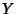## Homology (Topology)

Historically, the term ``homology'' was first used in a topological sense by Poincaré.To him, it meant pretty much what is now called a Cobordism, meaning that a homology was thought of as a relation between Manifolds mapped into a Manifold. Such Manifolds form a homology when they form the boundary of a higher-dimensional Manifold inside the Manifold in question.

To simplify the definition of homology, Poincaré simplified the spaces he dealt with. He assumed that all the spaces he dealt with had a triangulation (i.e., they were ``Simplicial Complexes''). Then instead of talking about general ``objects'' in these spaces, he restricted himself to subcomplexes, i.e., objects in the space made up only on the simplices in the Triangulation of the space. Eventually, Poincaré's version of homology was dispensed with and replaced by the more general Singular Homology. Singular Homology is the concept mathematicians mean when they say ``homology.''

In modern usage, however, the word homology is used to mean Homology Group. For example, if someone says ``didby computing the homology of,'' they mean ``didby computing the Homology Groups of.'' But sometimes homology is used more loosely in the context of a ``homology in a Space,'' which corresponds to singular homology groups.

Singular homology groups of a Space measure the extent to which there are finite (compact) boundaryless Gadgets in that Space, such that these Gadgets are not the boundary of other finite (compact) Gadgets in that Space.

A generalized homology or cohomology theory must satisfy all of the Eilenberg-Steenrod Axioms with the exception of the Dimension Axiom.

See also Cohomology, Dimension Axiom, Eilenberg-Steenrod Axioms, Gadget, Homological Algebra, Homology Group, Simplicial Complex, Simplicial Homology, Singular Homology